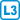## Characterization of the identity mapping

Let $$f: X \to X$$ and suppose that for all $$g: X\to X$$ it is true that $$f\circ g=g\circ f$$. Show that $$f$$ must be the identity mapping.

• #### Hint

If $$f$$ is not the identity mapping, look for a contradiction concerning $$g$$.

• #### Resolution

We first note that the identity mapping $$id$$ fulfills $$id\circ g=g=g\circ id$$.

We proceed by contradiction: assume that there exist distinct $$x$$ and $$y$$ such that $$f(x)=y$$.

If $$f(y)=y$$, we choose $$g$$ such that $$g(y)=x$$. Then $$(f\circ g)(y)=f(g(y))=f(x)=y$$, but $$(g\circ f)(y)=g(f(y))=g(y)=x$$, which is a contradiction.

If $$f(y)\ne y$$, we choose $$g$$ such that $$g(x)=g(y)=y$$. Then $$(f\circ g)(x)=f(g(x))=f(y)\ne y$$, but $$(g\circ f)(x)=g(f(x))=g(y)=y$$, which is a contradiction.